# Networks, Signals and Systems - Online Test

Q1. A dual trace oscilloscope is set to operate in the Alternate mode. The control input of the multiplexer used in the y-circuit is fed with a signal having a frequency equal to
Explaination / Solution:
No Explaination.

Q2. The r.m.s value of the current i(t) in the circuit shown below is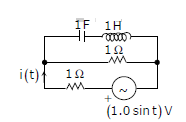Explaination / Solution: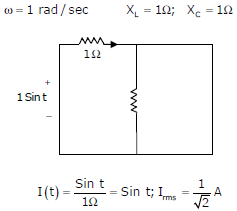Q3. A plane wave of wavelength λ is traveling in a direction making an angle 300 with positive x − axis and 900 with positive y − axis. The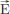field of the plane wave can be represented as (E is constant)
Explaination / Solution: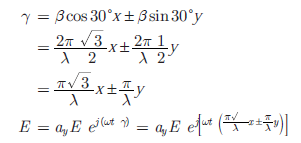Q4. The fourier series expansionof the periodic signal shown below will contain the following nonzero terms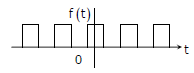Explaination / Solution:

⇒ it satisfies the half wave symmetry, so that it contains only odd harmonics.
⇒ It satisfies the even symmetry. So bn = 0

Q5. The voltage applied to a circuit is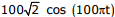volts and the circuit draws a current ofamperes. Taking the voltage as the reference phasor, the phasor representation of the current in amperes is
Explaination / Solution: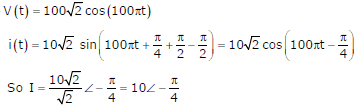Q6.
The steady state error of a unity feedback linear system for a unit step input is 0.1. The steady state error of the same system, for a pulse input r(t) having a magnitude of 10 and a duration of one second, as shown in the figure isExplaination / Solution:Q7.
In the circuit given below, the value of R required for the transfer of maximum power to the load having a resistance of 3W is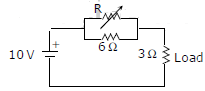Explaination / Solution: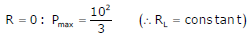Q8. Given two continuous time signals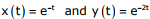which exist for t > 0, the convolution z(t) = x(t)* y(t) is
Explaination / Solution:Q9. A lossy capacitor Cx, rated for operation at 5 kV, 50 Hz is represented by an equivalent circuit with an ideal capacitor Cp in parallel with a resistor Rp. The value Cp is found to be 0.102 µF and the value of Rp = 1.25 MΩ . Then the power loss and tan ∂ of the lossy capacitor operating at the rated voltage, respectively,
Explaination / Solution:
No Explaination.

Q10. Let the Laplace transform of a function F(t) which exists for t > 0 be F1(s) and the Laplace transform of its delayed version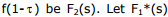be the complex conjugate of F1(s) with the Laplace variable set as s=σ + jw. If G(s) =, then the inverse Laplace transform of G(s) is
Explaination / Solution: